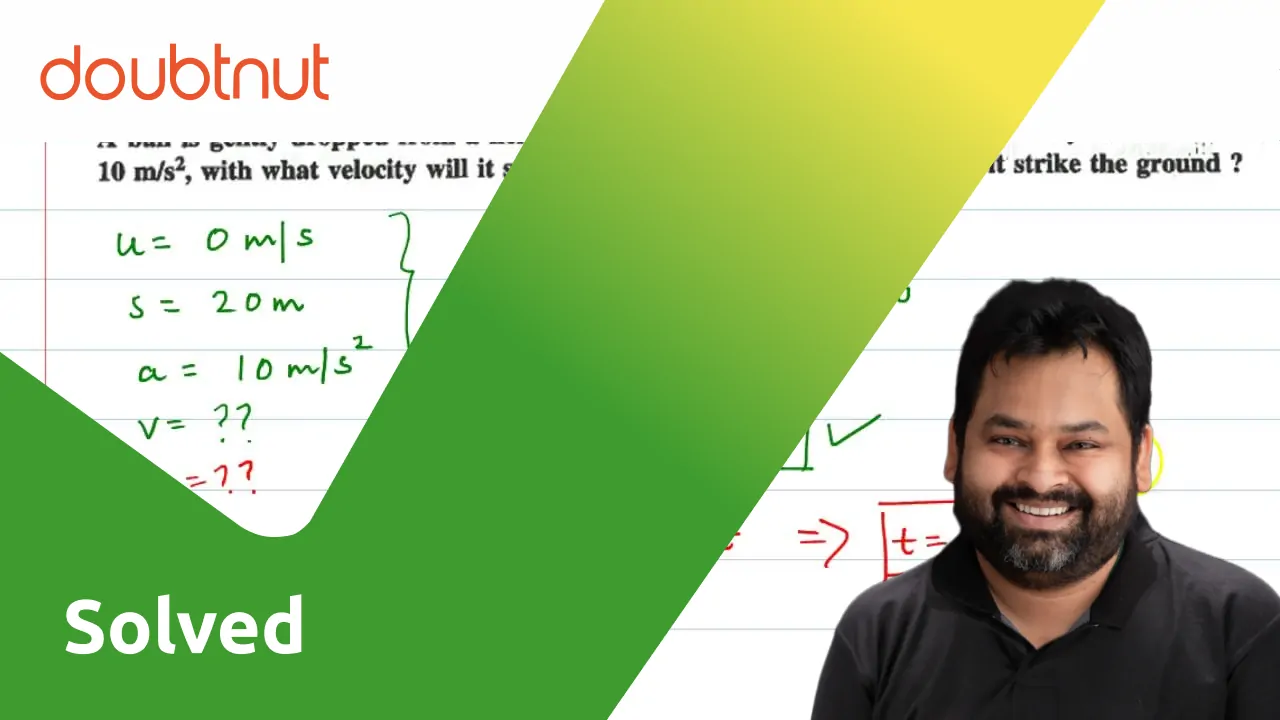Doubtnut App - Get 50 Lakh+ video solutions better than GoogleInstall Now

# A ball is gently dropped from a height of 20m. If its velocity increases uniformly at the rate of 10m/s2, with what velocity will it strike the ground ? After what time will it strike the ground ?

Text Solution
Verified by Experts

## Here, Height=distance=s=20m, acceleration, a=10m/s2 final velocity, v=?, time taken, t=? As the ball is gently dropped, its initial velocity, u=0 From v2−u2=2 as, v2=u2+2 as =0+2(10)×20=400 or v=√400=20m/s This is the velocity with which the ball will hit the ground. From v=u+at, 20=0+10t or t=2010=2s